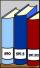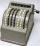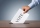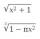Students at cinema

All pupils from the school went to the cinema for a film performance. The cinema has 400 seats, 2 rows of 20 seats remain unoccupied. 10 places were occupied by teachers. How many students were there?

Result

n =  350

Solution:Leave us a comment of this math problem and its solution (i.e. if it is still somewhat unclear...):Be the first to comment!Next similar math problems:

1. Flood waterFlood waters in some US village meant that the homes had to evacuate 364 people. 50 of them stayed at elementary schools, 59 them slept with their friends and others went to relatives. How many people have gone to relatives?
2. Expression 1What is 7+8-(5×2)+5-4+(6×(5-3)+6)-(8+10)-7+6?
3. What is missing (1000)What number is to add to get 1000?
4. Decimal expansionCalculate: 2 . 1 + 0 . 10 + 7 . 10000 + 4 . 1000 + 6 . 100 + 0 . 100000 =
5. Roman numerals 2+Add up the number writtens in Roman numerals. Write the results as a roman numbers.
6. Addition of Roman numbersAdded together and write as decimal number: LXVII + MLXIV
7. ProductResult of the product of the numbers 1, 2, 3, 1, 2, 0 is:
8. SubtractionTest what do you know about the subtraction of two numbers: a) make a difference if the minuend 4,307,288 and subtrahend is 472008 b) minuend is 4000 more than subtrahend. What's the difference? c) the difference is 38900 less than the minuend. What is th
9. Write decimalsWrite in the decimal system the short and advanced form of these numbers: a) four thousand seventy-nine b) five hundred and one thousand six hundred and ten c) nine million twenty-six
10. SummandOne of the summands is 145. The second is 10 more. Determine the sum of the summands.
11. OperationsSum of the numbers 1.01 and 3.35 multiply by the difference of numbers 6.69 and 1.39.
12. Voting400 employees cast their votes in a board member election that has only 2 candidates. 120 people vote for candidate A, while half of the remaining voters abstain. How many votes does candidate B receive?
13. Roman numerals 2-Subtract up the number written in Roman numerals. Write the results as Roman numbers.
14. Roman numerals +Add up the number writtens in Roman numerals. Write the results as a decimal number.
15. Roman numeralsWrite numbers written in Roman numerals as decimal.
16. With bracketCalculate (evaluate) simple mathematical expression with a negative numbers and a bracket: 13+15*5-2*(-6)
17. Product of the sum and differenceCalculate the product of the sum and difference of numbers -7 and -2.